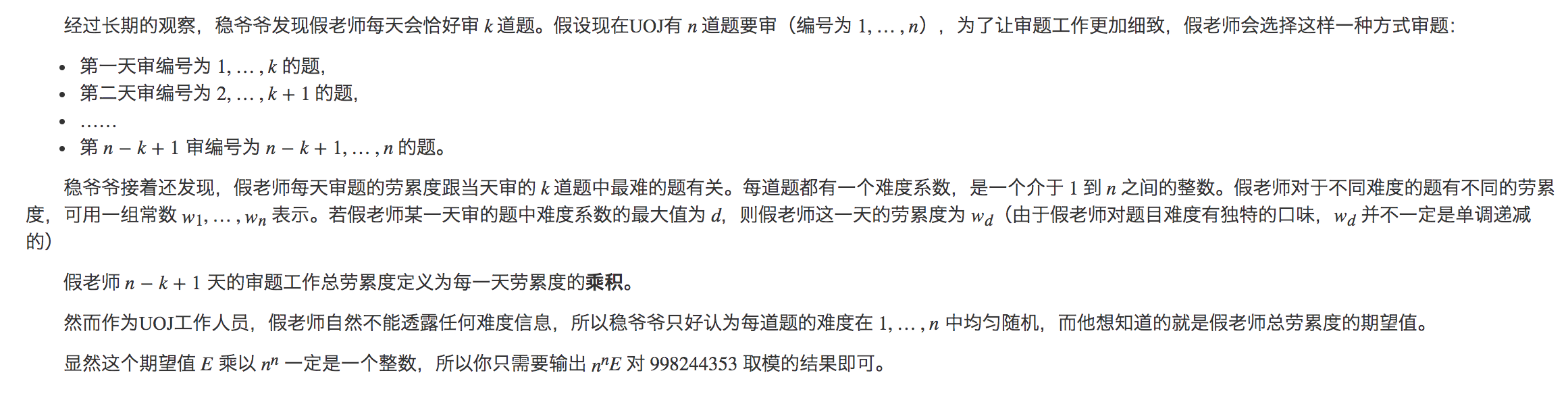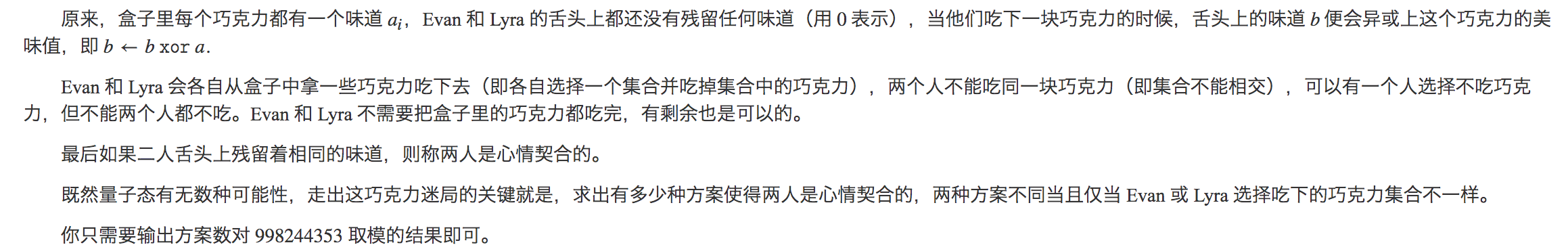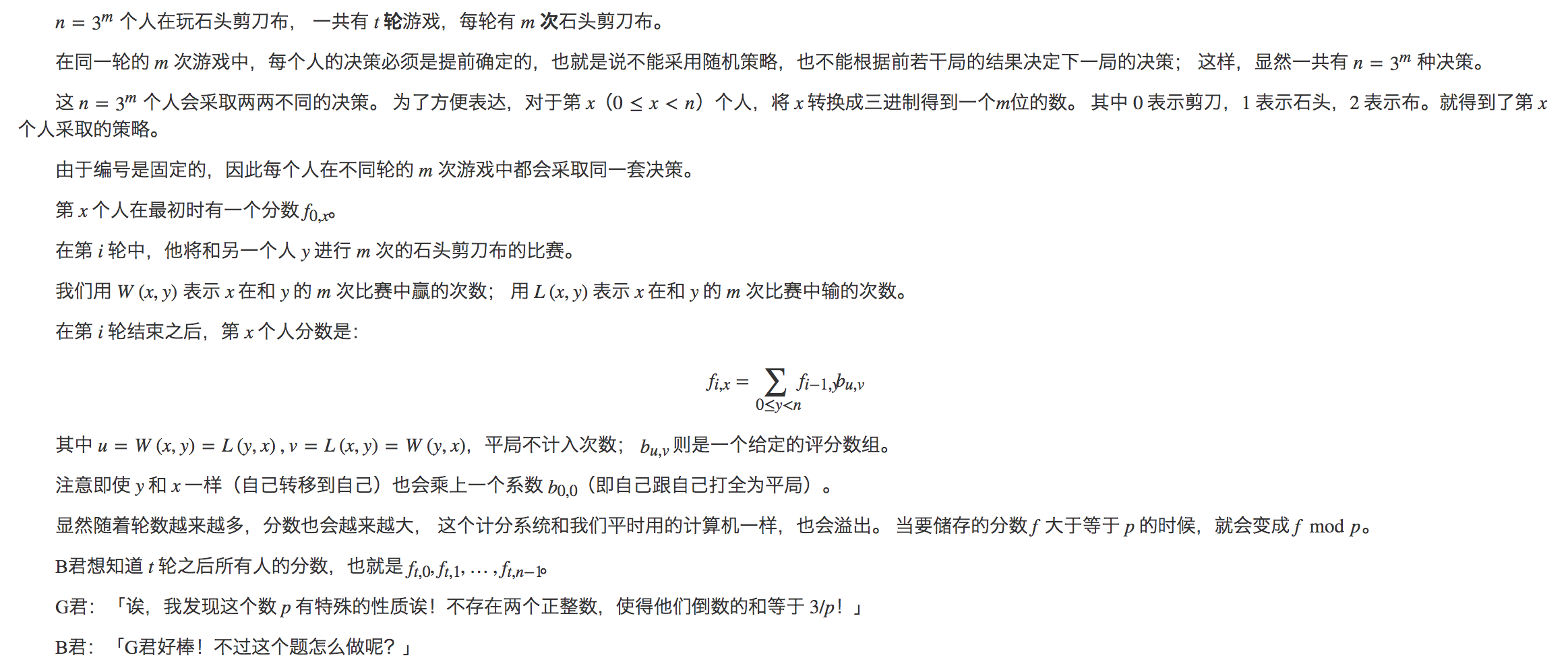## BZOJ4401 块的计数

#### 暴力 DP

DFS 自底向上计算，用 dp_u 表示到 u 为止，未被分块的节点个数。

## NOI模拟赛20190711B 三格骨牌(trominoes)before transformation after transformation image
0001 10000011 10100101 11000111 1110## LOJ575 「LibreOJ NOI Round #2」不等关系

f_{i, j} = \begin{cases} \displaystyle \sum_{k=1}^{i-1} f_{i-1, k} & (s_{i - 1} = \textrm{<}) \\ \displaystyle \sum_{k=i}^j f_{i-1, k} & (s_{i - 1} = \textrm{>}) \\ \end{cases}

dp_n = \begin{cases} 1 & (n = 0) \\ \displaystyle \sum_{j=0}^{i-1} [j = 0 \textrm{ or } s_j = \textrm{<}] (-1)^{cnt_{i-1} - cnt_j} \frac {dp_{j}} {(i - j)!} & (n > 0) \\ \end{cases}

## LOJ573 「LibreOJ NOI Round #2」单枪匹马

\left[\begin{matrix} a & 1 \\ 1 & 0 \\ \end{matrix}\right] \left[\begin{matrix} x \\ y \\ \end{matrix}\right] = \left[\begin{matrix} ax + y \\ x \end{matrix}\right]

\left[\begin{matrix} 0 & 1 \\ 1 & -a \\ \end{matrix}\right] \left[\begin{matrix} ax + y \\ x \\ \end{matrix}\right] = \left[\begin{matrix} x \\ y \\ \end{matrix}\right]

\begin{aligned} Ans_{l, r} &= \left[\begin{matrix} a_l & 1 \\ 1 & 0 \\ \end{matrix}\right] \left[\begin{matrix} a_{l + 1} & 1 \\ 1 & 0 \\ \end{matrix}\right] \cdots \left[\begin{matrix} a_r & 1 \\ 1 & 0 \\ \end{matrix}\right] \left[\begin{matrix} 1 \\ 0 \end{matrix}\right] \\ &= \left[\begin{matrix} 0 & 1 \\ 1 & -a_{l - 1} \\ \end{matrix}\right] \left[\begin{matrix} 0 & 1 \\ 1 & -a_{l - 2} \\ \end{matrix}\right] \cdots \left[\begin{matrix} 0 & 1 \\ 1 & -a_1 \\ \end{matrix}\right] \left[\begin{matrix} a_1 & 1 \\ 1 & 0 \\ \end{matrix}\right] \left[\begin{matrix} a_2 & 1 \\ 1 & 0 \\ \end{matrix}\right] \cdots \left[\begin{matrix} a_r & 1 \\ 1 & 0 \\ \end{matrix}\right] \left[\begin{matrix} 1 \\ 0 \end{matrix}\right] \end{aligned}

\begin{aligned} F_n &= \left[\begin{matrix} a_1 & 1 \\ 1 & 0 \\ \end{matrix}\right] \left[\begin{matrix} a_2 & 1 \\ 1 & 0 \\ \end{matrix}\right] \cdots \left[\begin{matrix} a_n & 1 \\ 1 & 0 \\ \end{matrix}\right] \\ G_n &= \left[\begin{matrix} 0 & 1 \\ 1 & -a_{n} \\ \end{matrix}\right] \left[\begin{matrix} 0 & 1 \\ 1 & -a_{n - 1} \\ \end{matrix}\right] \cdots \left[\begin{matrix} 0 & 1 \\ 1 & -a_1 \\ \end{matrix}\right] \end{aligned}

## LOJ6674 赛道修建

\clubsuit 是 OI 国马拉松协会的会长。有一天，他打算举办全国青少年马拉松奥林匹克联赛（National Olympiad in Marathon in Provinces, NOMP），于是准备在 OI 国中修建一条赛道。

OI 国有 n (n \leq 5 \times 10^4) 个城市，一共有 n - 1 条道路将它们连通。也就是说，OI 国的结构是一棵树。

\clubsuit 会选择一个城市作为起点，再选择一个城市作为终点，这样赛道的长度就等于起点到终点路径上的边数。由于 OI 国的群众非常懒，所以赛道长为 0 也是允许的。

## UOJ311 【UNR #2】积劳成疾## UOJ310 【UNR #2】黎明前的巧克力## UOJ272 【清华集训2016】石家庄的工人阶级队伍比较坚强## k 进制 FWT 学习笔记

\sum_{i \oplus j = k} A_i \times B_j = C_k

\begin{aligned} \operatorname{fwt}(A)[x] \times \operatorname{fwt}(B)[x] &= \operatorname{fwt}(C)[x] \\ \sum_{i=0}^n w(x, i) A_i \sum_{j=0}^n w(x, j) B_j &= \sum_{k=0}^n w(x, k) C_k \end{aligned}

\sum_{i \oplus j = k} w(x, i) A_i \times w(x, j) B_j = w(x, k) C_k

\forall i \oplus j = k, w(x, i) \times w(x, j) = w(x, k)